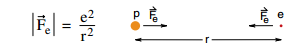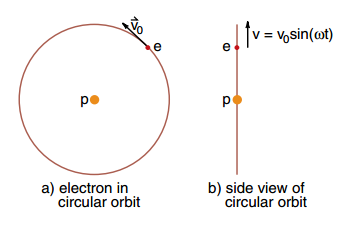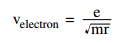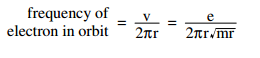Win up to 100% scholarship on Aakash BYJU'S JEE/NEET courses with ABNAT Win up to 100% scholarship on Aakash BYJU'S JEE/NEET courses with ABNAT

# Bohr’s Theory of Hydrogen Atoms

The hydrogen atom played a special role in the history of physics by providing the key that unlocked the new mechanics that replaced Newtonian mechanics. It started with Johann Balmer’s discovery in 1884 of a mathematical formula for the wavelengths of some spectral lines emitted by hydrogen.

The next step was Rutherford’s discovery of the atomic nucleus in 1912. After that, one came to know the basic structure of atoms – a positive nucleus surrounded by negative electrons. Within a year, Niels Bohr had a model of the hydrogen atom that explained the spectral lines. Bohr introduced a new concept, the energy level.  To explain the energy levels, Bohr developed a model in which the electron had certain allowed orbits, and the jump between energy levels corresponded to the electron moving from one allowed orbit to another.

## The Classical Hydrogen Atom

With Rutherford’s discovery of the atomic nucleus, it became clear that atoms consisted of a positively charged nucleus surrounded by negatively charged electrons that were held to the nucleus by an electric force. The simplest atom would be hydrogen consisting of one proton and one electron held together by a Coulomb force of magnitude.As shown in Equation 1, both the proton and the electron attract each other, but since the proton is 1836 times more massive than the electron, the proton should sit nearly at rest while the electron orbits around it.

Figure 1. The side view of circular motion is an up-and-down oscillationThe hydrogen atom is such a simple system, with known masses and known forces, that it should be a straightforward matter to make detailed predictions about the nature of the atom. We can use the orbit program, replacing the gravitational force GMm/r2 by e2/r2. We can predict that the electron moved in an elliptical orbit about the proton, obeying all of Kepler’s laws for orbital motion.

There is one important point we have to take into account in our analysis of the hydrogen atom that we do not have to worry about in our study of satellite motion. The electron is a charged particle, and accelerated charged particles radiate electromagnetic waves. Suppose, for example, the electron was in a circular orbit moving at an angular velocity ω, as shown in Figure (1a). If we were looking at the orbit from the side, as shown in Figure (1b), we would see an electron oscillating up and down with a velocity given by v = v0sin ωt.

If electrons oscillate up and down at a frequency, they produce radio waves of the same frequency. Thus, it is a prediction of Maxwell’s equations that the electron in the hydrogen atom should emit electromagnetic radiation, and the frequency of the radiation should be the frequency at which the electron orbits the proton.

For an electron in a circular orbit, predicting the motion is quite easy. If an electron is in an orbit of radius r, moving at a speed v, then its acceleration is directed towards the centre of the circle and has a magnitude,

$$\begin{array}{l}\vec{a} = \frac{v^2}{r}\end{array}$$

Using Equation 1 for the electric force and Equation 2 for the acceleration, which shows that the force is in the same direction as the acceleration (as indicated in Figure (2)), Newton’s second law gives,

$$\begin{array}{l}\frac{e^2}{r^2}=\frac{mv^2}{r}\end{array}$$

One factor of r cancels, and we can directly solve for the electron’s speed v to get v2 = e2/mr, orThe period of the electron’s orbit should be the distance 2πr travelled, divided by the speed v, or 2πr/v seconds per cycle, and the frequency should be the inverse of that or v/2πr cycles per second. Using Equation 4 for v, we getAccording to Maxwell’s theory, this should also be the frequency of the radiation emitted by the electron.

Test your Knowledge on Bohrs Theory Of Hydrogen Atoms